# The datasets used:¶

### - The second is from the World Health Organization: https://ourworldindata.org/coronavirus-source-data¶

In :
# Import libraries
import pandas as pd
import matplotlib.pyplot as plt
import numpy as np
from datetime import datetime
import seaborn as sns
import folium
import altair as alt
import csv
sns.set()

In :
# Read the first dataset into a pandas dataframe
df = pd.read_csv("covid_19_data.csv")

In :
print(df.shape)

(23158, 8)

In :
print(df.dtypes) # Columns

SNo                  int64
ObservationDate     object
Province/State      object
Country/Region      object
Last Update         object
Confirmed          float64
Deaths             float64
Recovered          float64
dtype: object

In :
# A list of all 50 states
state_list = ["Alabama","Alaska","Arizona","Arkansas","California","Colorado",
"Connecticut","Delaware","Florida","Georgia","Hawaii","Idaho","Illinois",
"Indiana","Iowa","Kansas","Kentucky","Louisiana","Maine","Maryland",
"Massachusetts","Michigan","Minnesota","Mississippi","Missouri","Montana",
"Nebraska","Nevada","New Hampshire","New Jersey","New Mexico","New York",
"North Carolina","North Dakota","Ohio","Oklahoma","Oregon","Pennsylvania",
"Rhode Island","South Carolina","South Dakota","Tennessee","Texas","Utah",
"Vermont","Virginia","Washington","West Virginia","Wisconsin","Wyoming"]

In :
# Here we iterate through the states extracting the confirmed cases, deaths, and recovered cases for each date
# in the dataset. We then append each row to a csv file and print out the information we extracted.
total_us_confirmed = 0
total_us_deaths = 0
total_us_recovered = 0
date = ""

states_with_deaths = []

for current_state in state_list:
current_df = df[np.logical_and(df['Province/State'] == current_state, df['Country/Region'] == 'US' )]

date = current_df.max().ObservationDate
total_us_confirmed += current_df.max().Confirmed
total_us_recovered += current_df.max().Recovered
total_us_deaths += current_df.max().Deaths

if(current_df.max().Deaths > 0):
states_with_deaths.append(current_state + ' (' + str(current_df.max().Deaths) + ')')

add_csv = [date, current_state, current_df.max().Confirmed, current_df.max().Deaths, current_df.max().Recovered]

with open(r'my_data.csv', 'a') as f:
writer = csv.writer(f)
writer.writerow(add_csv)

print("AS OF " + date + ":")
print("Total US confirmed: " + str(total_us_confirmed))
print("Total US deaths: " + str(total_us_deaths))
print("Total US recovered: " + str(total_us_recovered))

death_percent = total_us_deaths / total_us_confirmed * 100
recover_percent = total_us_recovered / total_us_confirmed * 100

print("Percent of confirmed cases that have died: " + "%.2f" % death_percent + "%")
print("Percent of confirmed cases that have recovered: " + "%.2f" % recover_percent + "%")

print("\nStates with deaths: ")
i = 0
for state in states_with_deaths:
if len(states_with_deaths) - 1 != i:
state+=', '
print(state, end=' ')
i += 1

AS OF 05/09/2020:
Total US confirmed: 1300888.0
Total US deaths: 78363.0
Total US recovered: 17.0
Percent of confirmed cases that have died: 6.02%
Percent of confirmed cases that have recovered: 0.00%

States with deaths:
Alabama (390.0),  Alaska (10.0),  Arizona (532.0),  Arkansas (88.0),  California (2687.0),  Colorado (967.0),  Connecticut (2932.0),  Delaware (221.0),  Florida (1715.0),  Georgia (1403.0),  Hawaii (17.0),  Idaho (67.0),  Illinois (3349.0),  Indiana (1490.0),  Iowa (252.0),  Kansas (174.0),  Kentucky (304.0),  Louisiana (2267.0),  Maine (64.0),  Maryland (1614.0),  Massachusetts (4840.0),  Michigan (4530.0),  Minnesota (558.0),  Mississippi (421.0),  Missouri (494.0),  Montana (16.0),  Nebraska (91.0),  Nevada (313.0),  New Hampshire (131.0),  New Jersey (9116.0),  New Mexico (191.0),  New York (26612.0),  North Carolina (551.0),  North Dakota (35.0),  Ohio (1331.0),  Oklahoma (270.0),  Oregon (127.0),  Pennsylvania (3779.0),  Rhode Island (418.0),  South Carolina (330.0),  South Dakota (34.0),  Tennessee (242.0),  Texas (1066.0),  Utah (66.0),  Vermont (53.0),  Virginia (827.0),  Washington (921.0),  West Virginia (52.0),  Wisconsin (398.0),  Wyoming (7.0) 
In :
# We then turn the csv file that was just created into a pandas dataframe and print out the last 5 entries to make
# sure it read in properly.
my_data_df = pd.read_csv('my_data.csv')
my_data_df.drop_duplicates(inplace = True)
my_data_df.to_csv('my_data.csv', index=False)
my_data_df.tail()

Out:
Date State Confirmed Deaths Recovered
2241 05/09/2020 Virginia 23196.0 827.0 0.0
2242 05/09/2020 Washington 16674.0 921.0 1.0
2243 05/09/2020 West Virginia 1323.0 52.0 0.0
2244 05/09/2020 Wisconsin 9939.0 398.0 1.0
2245 05/09/2020 Wyoming 653.0 7.0 0.0
In :
# Here we read in a csv file of the latitude/longitude of each state
lat_lng_df = pd.read_csv('lat_lng.csv')
lat_list = lat_lng_df['Lat'].tolist()
lng_list = lat_lng_df['Lng'].tolist()


## Next we take a look at the data in our newly created dataframe. You can click on each tooltip to see a bar graph of the current cases since 3/10/2020.¶

In :
# Iterate through states/coordinates and populate map
m = folium.Map(location=[40, -102], zoom_start=4)
i = 0
for state in state_list:
tmp_data = my_data_df[np.logical_and(my_data_df['State'] == state, my_data_df['Deaths']>=0 )]
tmp_data = tmp_data[["Date", "Confirmed"]]
tmp_data = tmp_data.iloc[::3]

chart = alt.Chart(tmp_data).mark_line().encode(x='Date', y='Confirmed')
vis1 = chart.to_json()

folium.Marker(
location= [lat_list[i],  lng_list[i]],
radius=20,
fill=True,
fillOpacity=1.0,
opacity=1.0,
tooltip=state,
popup=folium.Popup(max_width=600).add_child(folium.VegaLite(vis1, width=500, height=300)),
).add_to(m)

i += 1

m

Out:

# ----------------------------------------------------------------------------------------¶

In :
# Read the second dataset into a pandas dataframe
new_df = pd.read_csv('full_data.csv')
new_df = new_df.fillna(0) # replace all nan values with 0
new_df['date'] = pd.to_datetime(new_df['date']) # make sure dates are in proper format
new_df.drop_duplicates(inplace = True) # Remove duplicates

In :
new_df.shape

Out:
(16246, 6)
In :
new_df.dtypes # Columns

Out:
date            datetime64[ns]
location                object
new_cases                int64
new_deaths               int64
total_cases              int64
total_deaths             int64
dtype: object
In :
# Function that takes a country name and makes a graph
def country_plot(new_df, country_name):
temp_df = new_df[np.logical_and(new_df['location'] == country_name, new_df['total_cases']>0 )]

start_date = temp_df.min().date
end_date = temp_df.max().date

mask = (temp_df['date'] > start_date) & (temp_df['date'] <= end_date)
temp_df = temp_df.loc[mask]

title = country_name + " Coronavirus Deaths/Confirmed Cases"

plt.title(title, fontsize=20)

plt.plot(temp_df.date, temp_df.total_cases, label='Confirmed')
plt.plot(temp_df.date, temp_df.total_deaths, label='Deaths')

date_str = str(start_date.date()) + " through " + str(end_date.date())

plt.xlabel(date_str, fontsize=15)
plt.ylabel('Deaths/Confirmed', fontsize=15)

plt.gcf().autofmt_xdate()
plt.legend(fontsize=12)

fig = plt.gcf()
fig.set_size_inches(8, 5)
plt.xticks([])

plt.show()


## United States cases:¶

In :
country_plot(new_df, 'United States')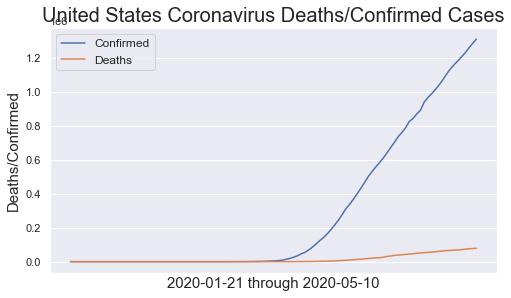## Italy cases:¶

In :
country_plot(new_df, 'Italy')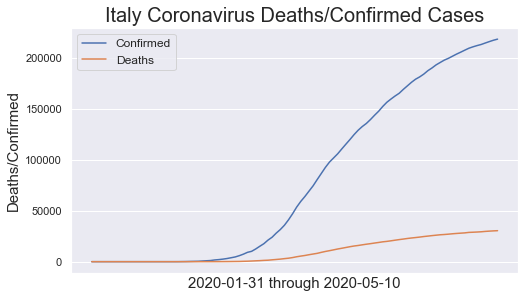## Iran cases:¶

In :
country_plot(new_df, 'Iran')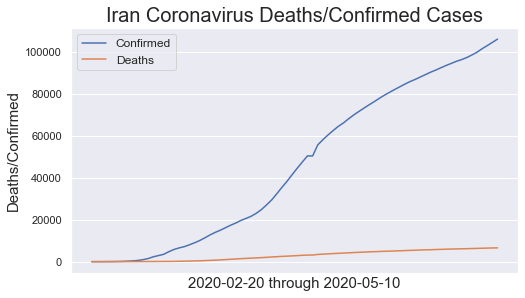## South Korea cases:¶

In :
country_plot(new_df, 'South Korea')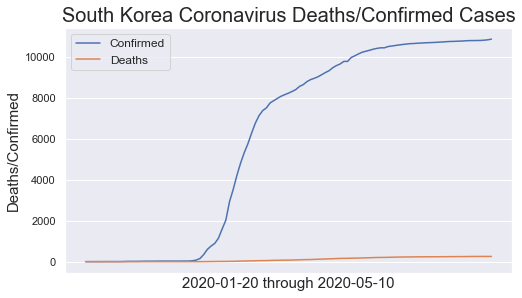## China cases:¶

In :
country_plot(new_df, 'China')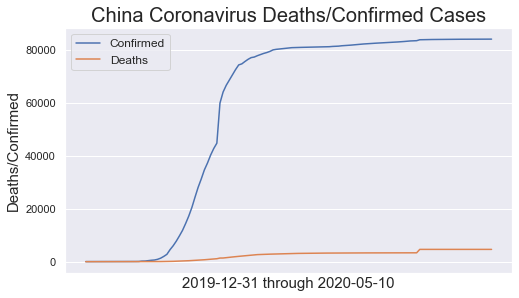In [ ]: